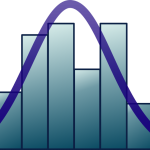# Quantitative Methods in Marine Science

University
Module
Teacher
Semester
Semester 1
Credits
6

Numerical tools help to ask scientific questions more efficiently and extract appropriate answers. This course will introduce students to many basic techniques in data analysis and numerical modelling, to help them summarize a problem in mathematical terms, plan experiments or field sampling campaigns, and gather insights from the data collected.

Students will learn how to identify sources of variation in biological data and decide on sampling/experimental units and replicates. Major inferential statistical and data exploration techniques will be taught. Numerical models will be introduced as a way to simplify and formalize a system. A programming language (R) will be used to apply all those techniques. Students will learn:

•   how to use computer code to read and manipulate data, to implement statistical tests or dynamical models
•   how to efficiently plan an experiment or field sampling campaign
•   how to choose an appropriate data analysis technique
•   how to interpret the output of basic inferential statistics
•   how to represent data and model output graphically